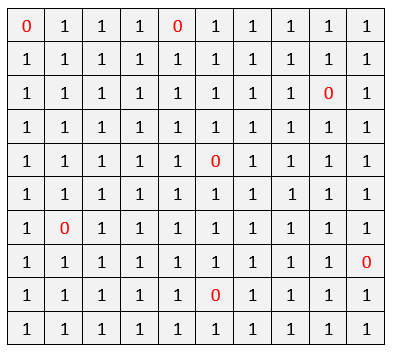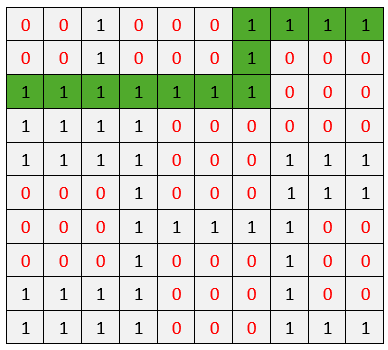# Find shortest safe route in a field with sensors present

Given a rectangular field with few sensors present on it, cross it by taking the shortest safe route without activating the sensors.

The rectangular field is given in the form of M x N matrix and we need to find the shortest path from any cell in first column to any cell in the last column of the matrix. The sensors are marked by value 0 in the matrix and all its 8 adjacent cells can also activate the sensors. The path can only be constructed out of cells having value 1 and at any given moment, we can only move one step in one of the four directions. The Valid moves are:

Go Up: (x, y) –> (x – 1, y)
Go Left: (x, y) –> (x, y – 1)
Go Down: (x, y) –> (x + 1, y)
Go Right: (x, y) –> (x, y + 1)

For example, consider below matrixThe shortest safe path has length of 11 and the route is marked in greenThe idea is to use BFS as it is a Shortest Path problem. Below is the complete algorithm.

1. Create a queue and enqueue every safe cell of first column and set their
distance as 0 from source (itself). Also mark them as visited as we push
them to the queue.

2. do till queue is not empty

a) Pop front node from the queue

b) If the popped node is destination node (last column), return its distance

c) else for each of 4 adjacent cells of current cell, we enqueue each valid
cell into the queue with +1 distance and mark them as visited

3. If all the nodes in the queue is processed and destination is not reached,
then return false

We can find all the possible locations we can move to from the given location by using the array that stores the relative position of movement from any location. For example, if the current location is
(x, y), we can move to (x + row[k], y + col[k]) for 0 <= k < 4 using below array.

int row[] = { -1, 0, 0, 1 };
int col[] = { 0, -1, 1, 0 };

So, from position (x, y), we can move to:

(x – 1, y)
(x, y – 1)
(x, y + 1)
(x + 1, y)

Note that in BFS, all cells having shortest path as 1 are visited first, followed by their adjacent cells having shortest path as 1 + 1 = 2 and so on.. so if we reach any node in BFS, its shortest path = shortest path of parent + 1. So, the first occurrence of the destination cell gives us the result and we can stop our search there. It is not possible that the shortest path exists from some other cell for which we haven’t reached the given node yet. If any such path was possible, we would have already explored it.

## C++

Output:

The shortest safe path has length of 11

## Java

Output:

The shortest safe path has length of 11

The time complexity of above solution is O(MN).(2 votes, average: 5.00 out of 5)Loading...

Please use our online compiler to post code in comments. To contribute, get in touch with us.
Like us? Please spread the word and help us grow. Happy coding 🙂